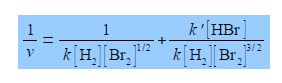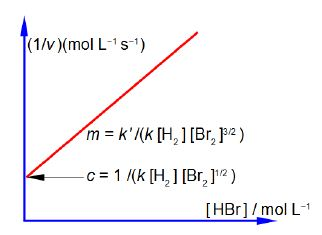# What are Kinetics of Complex Chemical Reaction?

The reaction at high temperature between H2 and Br2 molecules in the gas phase gives HBr molecules.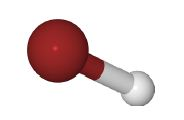HBr, H= gray, Br= red

Studying the chemical kinetics of this reaction, chemists have found that the rate of formation of HBr molecules v is as given below where k and k’ are parameters which only depend upon the temperature of the reaction. The symbol v for rate of reaction (“vee” as in velocity) should not be confused with the Greek letter v (“nu”) with the context removing any confusion. The concentration of e.g. H2 is [H2] mol L-1 but for clarity reaction rate equations are usually written without the units being explicitly shown.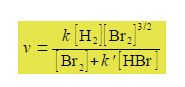Firstly, rearrange this equation for plotting as a straight line graph of 1/v against [HBr] by first taking reciprocals of both sides. Then simplify the right hand side of the new equation to get the final form which is ready for a linear plot. Second, what are the gradients and intercepts of this equation?

Solution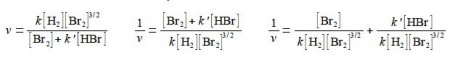We have taken reciprocals on both sides of the equation and then the right hand term can be split into two terms with the same denominator. The first of these two terms may then be simplified further by cancelling out [Br2] top and bottom to give a straight line graph (y = c + mx) of 1/v against [HBr] as in below Figure.